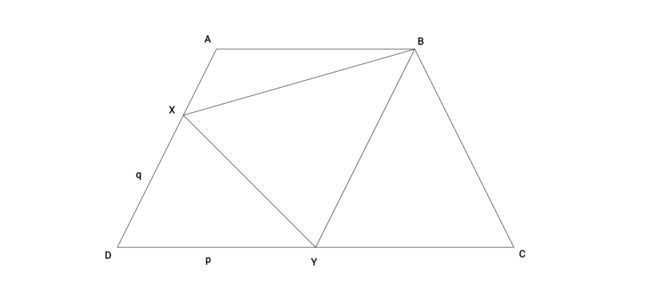# Triangle-Ception II

Geometry Level 5Quadrilateral $ABCD$ is an isosceles trapezoid with $AB=6,BC=6,CD=12,AD=6$.

Points $X$ and $Y$ are chosen on sides $AD$ and $DC$ respectively, such that $DX=q$ and $DY=p$.

If the area of triangle $XYB$ is $11\sqrt{3}$, the minimum value of $p-q$ can be expressed in the form $m\sqrt{n}$, where $n$ is not divisible by the square of any prime. Find $m+n$.

×